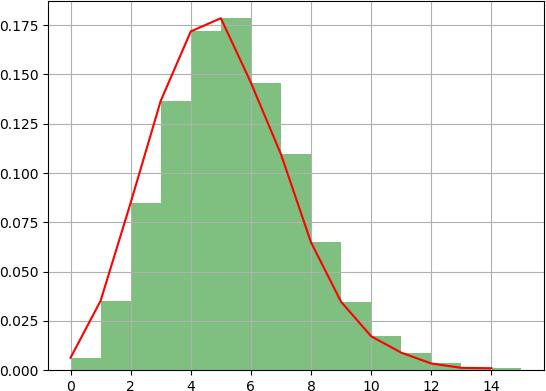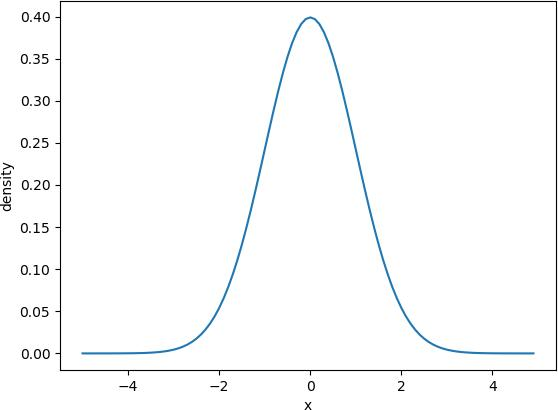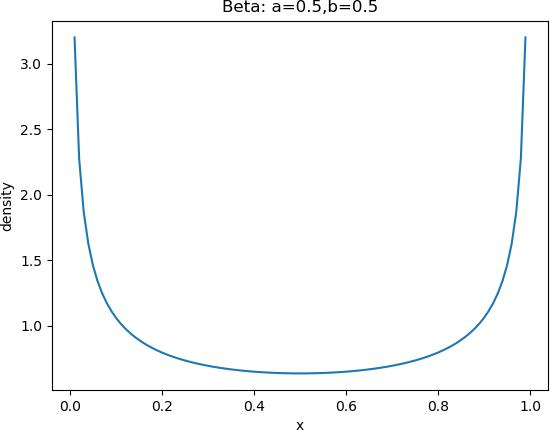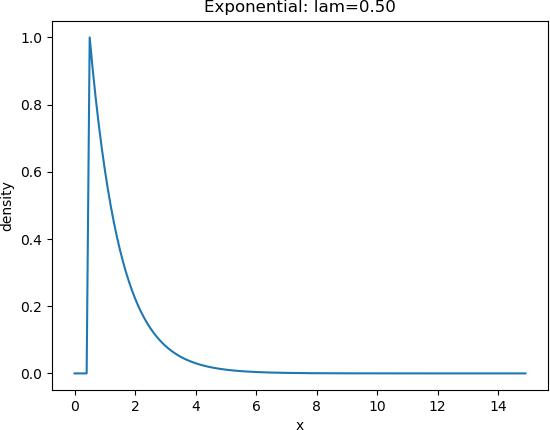020-38289118# {HCNA-AI 数学知识}之概率论内容介绍

3.1 概率论内容介绍

3.1.1 概率论介绍

3.1.2 实验介绍

3.2 概率论内容实现

>>> import numpy as np

>>> import scipy as sp

3.2.1 均值实现

# 数据准备

>>> b = [1,3,5,6]

>>> ll = [[1,2,3,4,5,6],[3,4,5,6,7,8]]

# 代码输入：

>>> np.mean(b)

# 结果输出：

>>> 3.75

>>> np.mean(ll) #全部元素求均值

# 结果输出：

>>> 4.5

>>> np.mean(ll,0) #按列求均值

# 结果输出：

>>> [2. 3. 4. 5. 6. 7.]

>>> np.mean(ll,1) #按行求均值

# 结果输出：

>>> [3.5 5.5]

3.2.2 方差与标准差实现

# 数据准备

>>> b=[1,3,5,6]

>>> ll=[[1,2,3,4,5,6],[3,4,5,6,7,8]]

# 求方差：

# 代码输入：

>>> np.var(b)

# 结果输出：

>>> 3.6875

# 代码输入：

>>> np.var(ll)

# 结果输出：

>>> 3.6874999999999996

# 代码输入：

>>> np.var(ll,1)) #第二个参数为 1，表示按行求方差

# 结果输出：

>>> [2.91666667 2.91666667]

3.2.3 标准差实现

# 数据准备

>>> b=[1,3,5,6]

>>> ll=[[1,2,3,4,5,6],[3,4,5,6,7,8]]

# 代码输入：

>>> np.std(b)

# 结果输出：

>>> 1.920286436967152

# 代码输入：

>>> np.std(ll)

# 结果输出：

>>> 1.9790570145063195

3.2.4 协方差实现

# 数据准备

>>> b=[1,3,5,6]

# 代码输入：

>>> np.cov(b)

# 结果输出：

>>> 4.916666666666666

3.2.5 相关系数

# 数据准备

>>> vc=[1,2,39,0,8]

>>> vb=[1,2,38,0,8]

# 代码输入：

>>> np.corrcoef(vc,vb)

# 结果输出：

>>> 4.916666666666667

# 代码输入：

>>> np.mean(np.multiply((vc-np.mean(vc)),(vb-np.mean(vb))))/(np.std(vb)*np.std(vc))

# 结果输出：

>>> 4.916666666666666

3.2.6 二项分布实现

# 代码输入：

from scipy.stats import binom, norm, beta, expon import numpy as np

import matplotlib.pyplot as plt

binom_sim = binom.rvs(n=10, p=0.3, size=10000) print('Data:',binom_sim)

print('Mean: %g' % np.mean(binom_sim)) print('SD: %g' % np.std(binom_sim, ddof=1)) plt.hist(binom_sim, bins=10, normed=True) plt.xlabel(('x'))

plt.ylabel('density') plt.show()

# 结果输出：

Data: [2 4 3 ... 3 4 1]

Mean: 2.9821

SD: 1.43478

# 分布图如下：3.2.1 泊松分布实现

# 代码输入：

import numpy as np

import matplotlib.pyplot as plt

x = np.random.poisson(lam=5, size=10000) pillar = 15

a = plt.hist(x, bins=pillar, normed=True, range=[0, pillar], color='g', alpha=0.5) plt.plot(a[0:pillar], a, 'r')

plt.grid() plt.show()

# 分布图如下：3.2.1 正态分布

from scipy.stats import norm import numpy as np

import matplotlib.pyplot as plt

mu = 0

sigma = 1

x = np.arange(-5, 5, 0.1)

y = norm.pdf(x, mu, sigma) plt.plot(x, y)

plt.xlabel('x') plt.ylabel('density') plt.show()

# 分布图如下：3.2.1 beta 分布

beta 分布是一个取值在 [0, 1] 之间的连续分布，它由两个形态参数α和β的取值所刻画。

# 代码输入：

from scipy.stats import beta import numpy as np

import matplotlib.pyplot as plt

a = 0.5

b = 0.5

x = np.arange(0.01, 1, 0.01) y = beta.pdf(x, a, b) plt.plot(x, y)

plt.title('Beta: a=%.1f,b=%.1f' % (a, b)) plt.xlabel('x')

plt.ylabel('density') plt.show()

# 分布图如下：3.2.1 指数分布

# 代码输入：

from scipy.stats import expon import numpy as np

import matplotlib.pyplot as plt

lam = 0.5

x = np.arange(0, 15, 0.1) y = expon.pdf(x, lam) plt.plot(x, y)

plt.title('Exponential: lam=%.2f' % lam) plt.xlabel('x')

plt.ylabel('density') plt.show()

# 分布图如下：十年老品牌
QQ咨询：450959328 微信咨询：togogozhong 咨询电话：020-38289118 咨询网站客服：在线客服